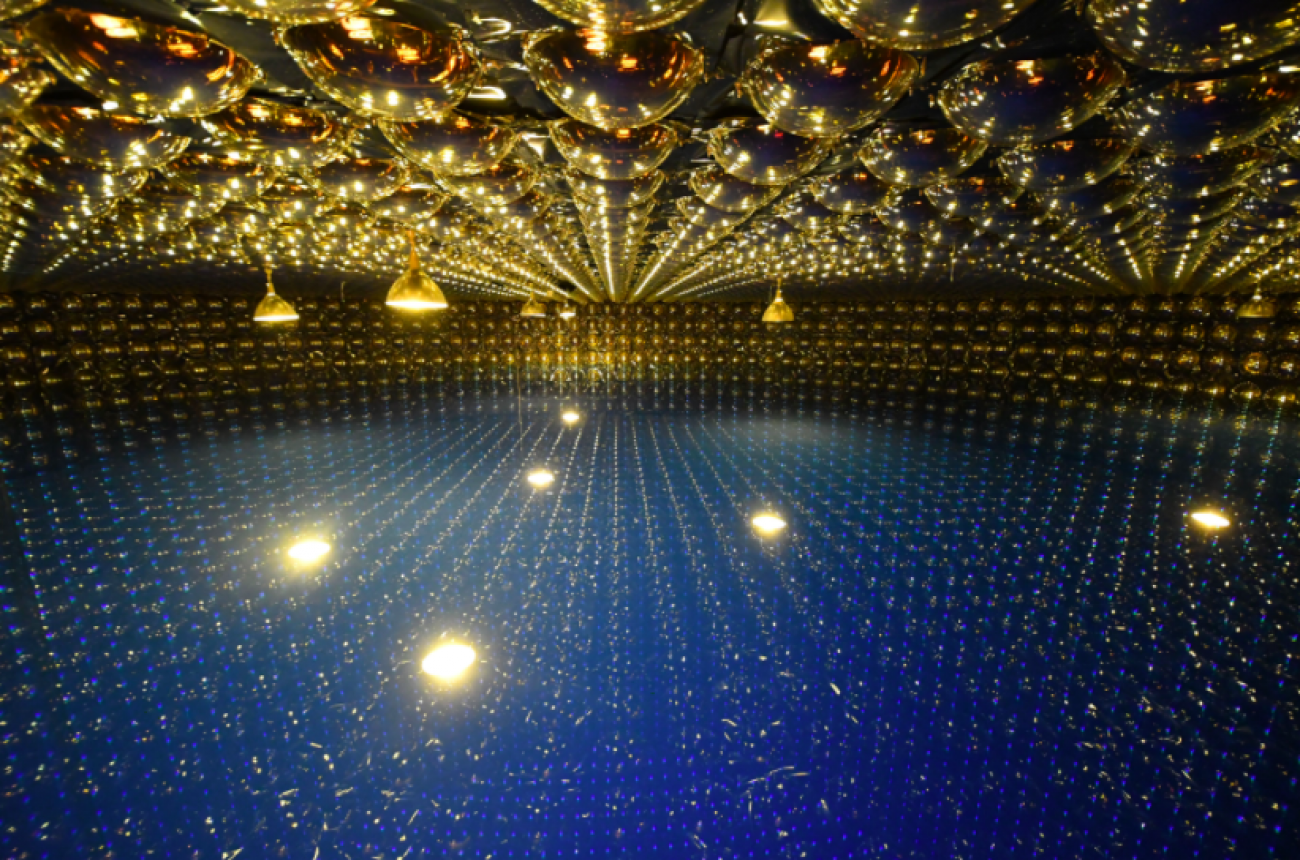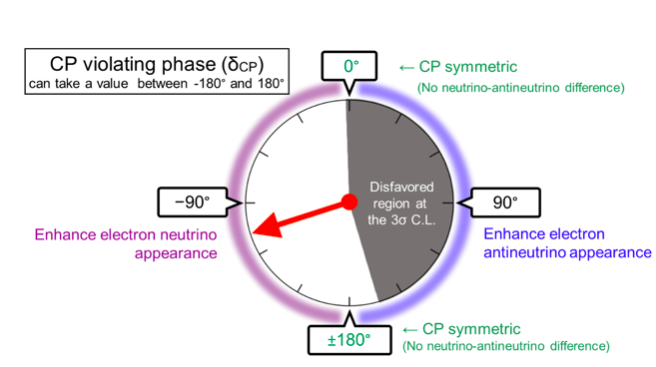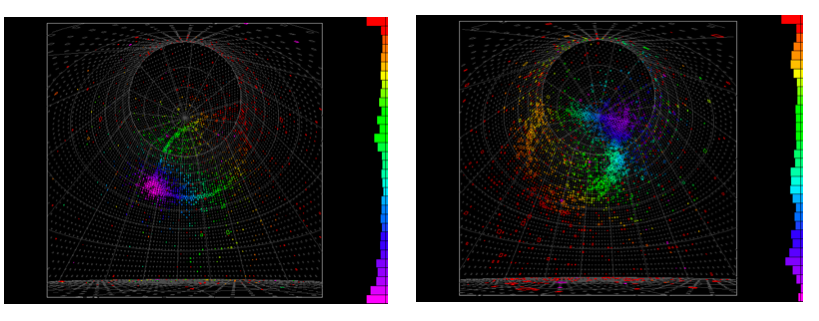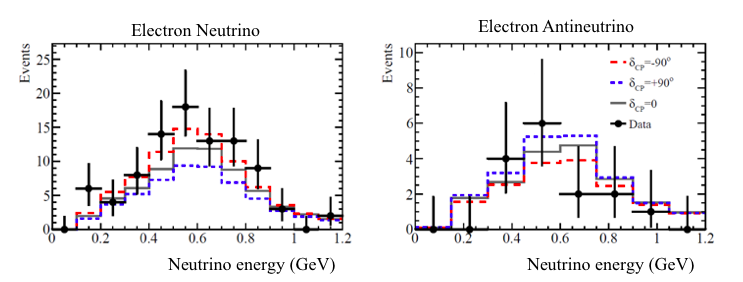# T2K Neutrino Oscillation ResultsThe T2K collaboration studies neutrino oscillations. These oscillations arise from quantum mechanical interference caused by the fact that every neutrino of the type electron, muon, or tau is a combination of three neutrino mass states. The neutrino type is determined by the associated heavy lepton (electron, muon, or tau) in the interaction, and has three paths to travel from the production to the interaction points, each associated with one of the neutrino mass states. The neutrinos travel as a superposition of these three states, each with a different mass and speed, producing interference patterns. Experimentally, this quantum mechanical interference is measured by looking at the appearance of types of neutrinos at the interaction point different from the ones that were produced. Particularly in T2K, we look for the transformation of muon neutrinos into electron neutrinos. The CP phase induces differences in the oscillation for the neutrinos and its antiparticles, the antineutrinos. In T2K, we have measured the oscillation parameters for neutrinos and antineutrinos and from the difference we can infer the value of the CP violation phase δCP. The latest result from T2K excludes about half of the possible values of δCP, and in particular the positive values of the phase angle are disfavoured with a confidence level of 99.7%. Following the convention where possible values of δCP run from -180 degrees to 180 degrees we have excluded values from -1.7 degrees to 164.6 degrees at 3 sigma confidence level.Figure 1: The arrow indicates the value most compatible with the data. The grey region is disfavoured at 99.7% (3σ) confidence level. Nearly half of the possible levels are excluded (Credit: T2K collaboration).

T2K produces neutrinos by colliding protons on a graphite target. These collisions produce charged pions and kaons that disintegrate inside a large decay volume producing neutrinos and antineutrinos.  The experiment can produce both neutrinos and antineutrinos simply by focusing or defocusing positively charged pions and negatively charged pions using a set of magnetic "horns" that act as a magnetic lens. The positive pions produce neutrinos during their disintegration and negative pions produce antineutrinos. The neutrino flavor composition and energy distributions are measured in a near detector before the oscillation becomes a significant contribution. The near-site measurement can distinguish neutrinos from antineutrinos using a magnetized detector. The far detector is located at 295km, exactly at a distance that enhances the oscillation probability. The far detector is capable of distinguishing muon neutrinos from electron neutrinos but it is not sensitive to neutrinos vs antineutrinos. The relative composition between particles and antiparticles is given by the near detector measurement and experiments providing the measurement of pions and kaons in proton-graphite collisions.Figure 2: Event displays of candidate electron neutrino (left) and electron antineutrino (right) events observed in Super-K from the T2K neutrino beam. When an electron neutrino or antineutrino interacts with water, an electron or positron is produced. They emit a faint ring pattern of light, detected by about 11,000 photo-sensors. The colour in the displays represents the photon detection timing.

The number of events in the far detector is very small. The recently published paper relies on 115 electron neutrino events including the antineutrino sample. This data has been taken over 10 years, so the stability of the whole system, mainly the beam intensity and direction, is a critical item in the analysis. The other important point is the reconstruction of the energy of the neutrino. The neutrino beam has a broad spectrum and the oscillation is a phenomenon that depends on the energy. The energy reconstruction depends on the neutrino-nucleus interactions that are rather poorly known both experimentally and theoretically.   A good fraction of the effort of the collaboration is devoted to the understanding, development, and measurement of these cross-sections in close contact with nuclear theorists.  The last challenge is statistical analysis.  To perform the measurement of the oscillation, the collaboration handles more than 200 systematic parameters associated with neutrino flux and cross-section uncertainties. These systematic uncertainties are constrained by the near detector and then propagated to the far detector to predict the flux of neutrinos in the absence of oscillations, which is by no means a trivial task. Three different analyses are carried out in parallel following the frequentist and Bayesian schools of statistics to check the correctness of the result.Figure 3: The observed electron neutrino (left) and electron antineutrino (right) candidate events with predictions for maximal neutrino enhancement (red, long dash) and maximum antineutrino enhancement (blues, short dash).

The result from T2K disfavours about half of the possible values of δCP, particularly the positive values of the phase angle are excluded with a confidence level of 99.7%. This is the first time we have constrained experimentally this fundamental parameter in the Standard Model. Another important feature of the T2K results is that the most probable solution is a value of the δCP that implies maximal violation of the CP symmetry in neutrinos. The fact that it can be maximal opens possible ways to understand the mechanism that differentiates neutrino mass states from flavor states. The last piece of information obtained from this result is a mild preference for a neutrino mass ordering, the so-called normal hierarchy.

Neutrino oscillations are, as far as we know, the only possibility to measure CP violation in neutrinos given present technology. Other theoretical options such as the measurement of lepton number violations using heavy leptons are very far from our actual technical capabilities.  So, the complementarity has to come from different experimental approaches while keeping the same experimental concept.  The potential differences may include different baselines, different neutrino energies, and different neutrino detection techniques. All these differences imply also different methods to reconstruct the energy of the neutrino associated with energy spectrum and detector technology. Even if these differences seem trivial, this type of complementarity is crucial to control some of the most challenging aspects of the measurement, the neutrino cross-sections, and to increase the sensitivity to the neutrino mass hierarchy that is enhanced by the modification of the oscillation when neutrinos cross the earth’s crust.

The future experiments, one in Japan and one in the US, will explore this complementarity exhaustively. The Japanese program, including the running T2K and the future HyperKamiokande, shows fewer matter effects, so the measurement of CP is less affected by it and neutrino hierarchy, and the neutrino energy reconstruction is based on the kinematic imbalance.  The  US program, including Nova and DUNE, has longer base-lines and larger matter effects, and utilizes the calorimetric approach to reconstruct the neutrino energy due to its slightly higher neutrino energy.  The DUNE experiment, the only one with a broad neutrino spectrum, will explore the measurement of two oscillation maxima occurring at two different neutrino energies. CP violation asymmetry has a distinct energy dependency at a fixed flight distance that can be exploited to improve measurements. A similar two maxima technique is also exploited by the proposal of H2KK. In this case, two detectors are located at different distances with a similar neutrino energy spectrum. Each one is sensitive to a different oscillation maximum and with different matter effects.